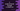# C program to create and iterate through a linked list### C program to create and iterate through a linked list :

In this C programming tutorial we will learn how to insert elements into a linked list and how to traverse through the linked list.

The linked list is an ordered list of elements, we call them node, where each node holds the address of its successor node. Each node will hold values and the address of the next node.

For example, suppose the linked list contains three nodes that hold values 1,2 and 3. In this case, the node containing value 1 will also contain the address of the second node, i.e. the node holding value 2.

In this example, we will learn how to create a simple linked list in C and how to insert values to the elements of the linked list.

The program will ask the user to the input value of each node, and then it will store the value in each node. Let’s take a look at the program :

### C program to implement linked list :

``````#include <stdio.h>
#include <stdlib.h>

//1
typedef struct node
{
int value;
struct node *next;
} node;

int main()
{
int length, i;

//2
printf("Enter size of the list : ");
scanf("%d", &length);

//3
struct node *headNode, *currentNode, *temp;

//4
for (i = 0; i < length; i++)
{
//5
currentNode = (node *)malloc(sizeof(node));

//6
printf("Enter element %d : ", (i + 1));
scanf("%d", &currentNode->value);

//7
if (i == 0)
{
headNode = temp = currentNode;
}
else
{
//8
temp->next = currentNode;
temp = currentNode;
}
}

//9
temp->next = NULL;

//10

//11
printf("Iterating through the elements of the linked list : \n");
while (temp != NULL)
{
//12
printf("%d \n", temp->value);
temp = temp->next;
}
}``````

### Explanation :

The commented numbers in the above program denote the step number below:

1. Each node of the linked list is defined by one structure. The structure name is node, and it can hold one integer value and address of next node.
2. Ask the user to enter the size of the linked list. Read and store it in variable length.
3. Create three variables to store the address of type node.
4. Start one for loop. Inside this loop, we will create each node for the list.
5. Create one node and store the address in currentNode.
6. Ask the user to enter the element for this node. Read and store it in the value of the node whose address is in currentNode.
7. Check if it is the first node in the loop or not. If first, store the same address in temp and headNode also.
8. If it is not the first node, set the value of currentNode to the next address of temp. This means, temp is linked to the currentNode.
9. After the loop is completed, set the value of next of temp as NULL.
10. Set the value of head to temp.
11. Now, iterate through the elements of the list using a while loop. If the current node is NULL, exit from the loop.
12. Print the value for each node and set the next address to current.

### Sample Output :

``````Enter size of the list : 5
Enter element 1 : 4
Enter element 2 : 2
Enter element 3 : 1
Enter element 4 : 9
Enter element 5 : 5
Iterating through the elements of the linked list :
4
2
1
9
5

Enter size of the list : 4
Enter element 1 : 1
Enter element 2 : 4
Enter element 3 : 6
Enter element 4 : 0
Iterating through the elements of the linked list :
1
4
6
0``````